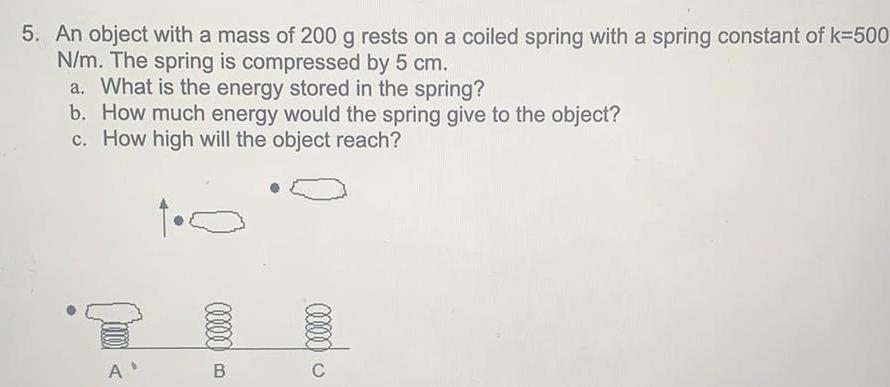Question:

# 5. An object with a mass of 200 g rests on a coiled spring with a spring constant of k=500 N/m. The spring is compressed by 5 cm. a. What is the energy stored in the spring? b. How much energy would the spring give to the object? c. How high will the

Last updated: 7/23/2022An object with a mass of 200 g rests on a coiled spring with a spring constant of k=500 N/m. The spring is compressed by 5 cm. a. What is the energy stored in the spring? b. How much energy would the spring give to the object? c. How high will the object reach?